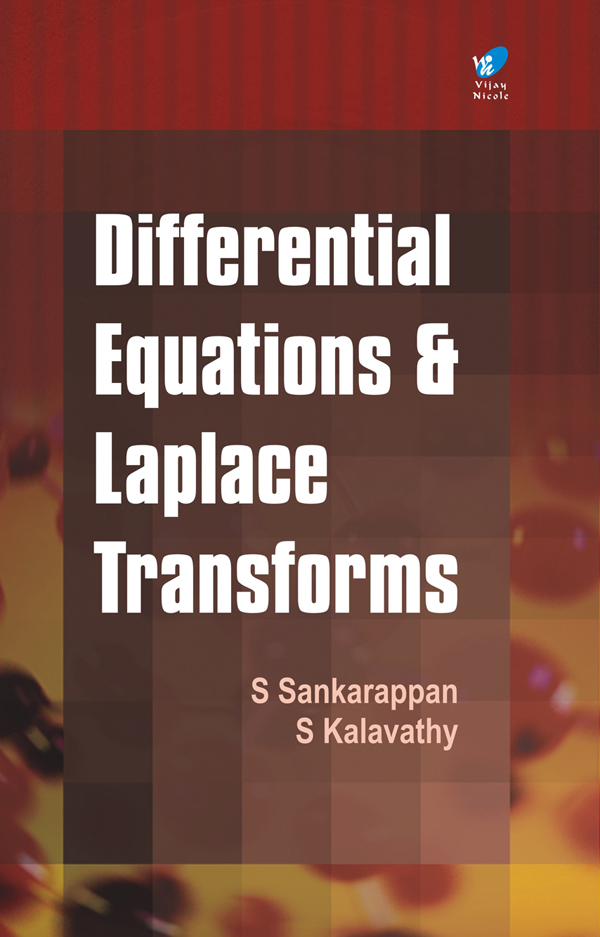Differential Equations and Laplace Transforms
Subject(s):
ISBN 9789394681002
Publication Date
Pages 302
Description

This book Differential Equations and Laplace Transforms, adopts a solved problems approach to the subject. Comprehensive, student-friendly, clear and concise, it is specifically designed to suit the requirements of undergraduate students of Mathematics

Cover
Title Page
Preface
Contents
Chapter 1 ----- First Order Differential Equation for Higher Order
1.1 Table of Integration Formulas
1.2 Order of the Differential Equation
1.3 Degree of the Differential Equation
1.4 First Order but of Higher Degree Differential Equations
1.5 Clairaut’s Form
Chapter 2 -----Ordinary Differential Equations-I
2.1 Ordinary Differential Equations
2.2 Solutions of the Equations with Constant Coefficients
2.3 Simultaneous Differential Equations
2.4 Method of Undetermined Coefficients for Finding the Particular Intergral
Chapter 3 ----- Ordinary Differential Equations-II
3.1 Linear Equations with Variable Coefficients
3.2 Solution by the Method of Variation of Parameters
Chapter 4 ----- Partial Differential Equations
4.1 Formation of PDE
4.1.1 Elimination of Arbitrary Constants
4.1.2 Elimination of Arbitrary Functions
4.2 First Order Nonlinear Partial Differential Equations
4.2.1 Singular Integral
4.2.2 General Integral
4.3 Standard Types
4.4 Equations Reducible To Standard Forms
4.5 Lagrange’s Linear Partial Differential Equation
4.5.1 Solutions of the Simultaneous Equations
4.6 Charpit’s Method
Chapter 5 ----- Laplace Transform
5.1 Definition
5.2 Existence of the Laplace transform
5.3 Laplace Transform of Standard Functions
5.4 First Shifting Theorem
5.5 Transform of Derivatives
5.6 Transforms of Integrals
5.7 Properties
5.8 Derivatives and Integrals of Transforms
5.9 Laplace Transform of Special Function
5.10 Second Shifting Theorem
5.11 Initial Value Theorem (I.V.T.)
5.12 Final Value Theorem (F.V.T.)
5.13 Inverse Laplace Transforms
5.14 Laplace Transform for Periodic Functions
5.15 Applications of Laplace transform in Linear Differential Equation
5.16 Simultaneous Differential Equations

S Kalavathy is Professor, Department of Mathematics, RMD Engineering College, Chennai. She has over 23 years of experience in teaching Mathematics and has written several books including best sellers on Engineering Mathematics and Operational Research.